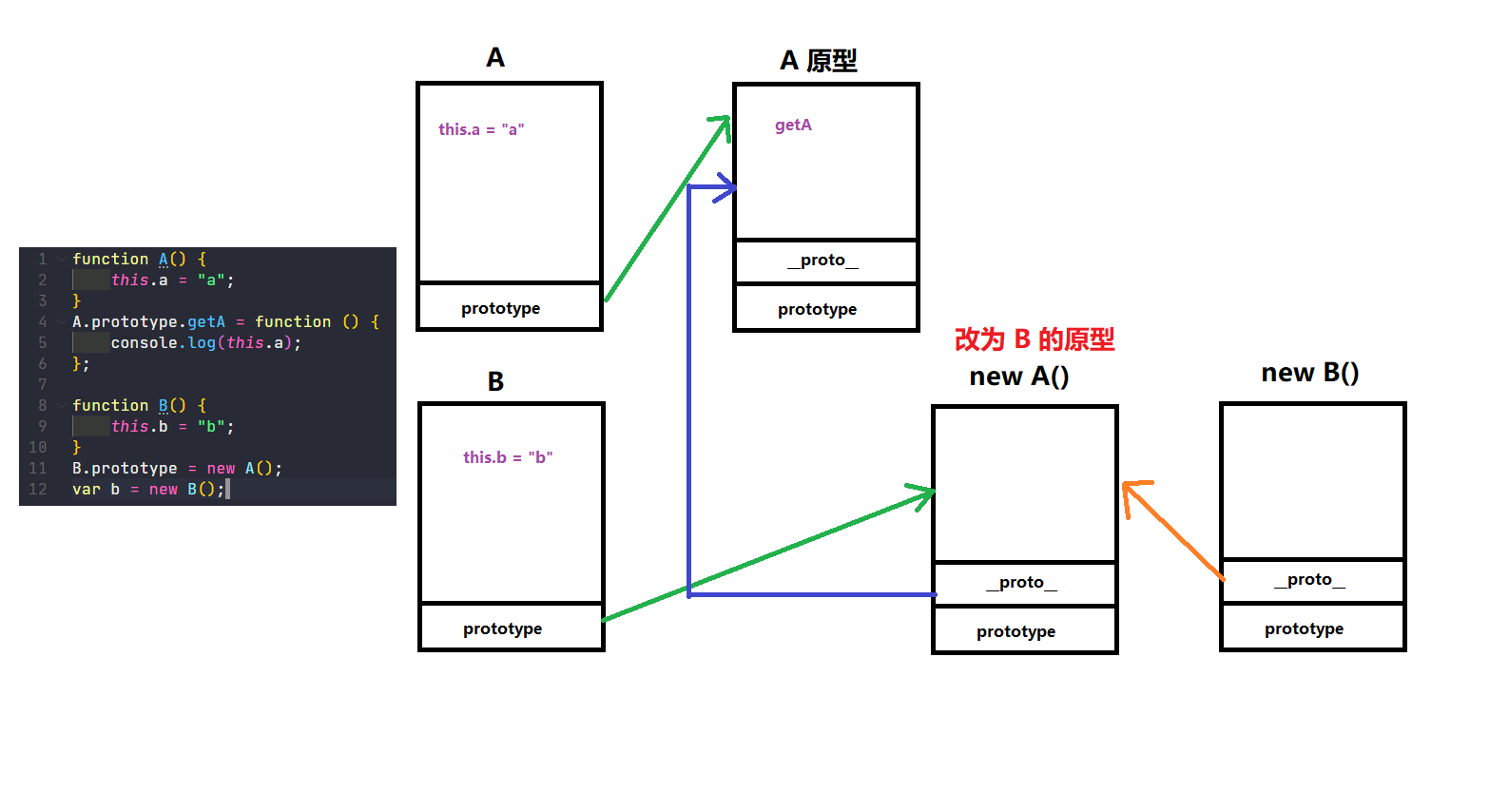JS 继承的四种方式

31 篇文章 0 订阅

类三大特性

JS 本身是基于面向对象开发的编程语言。类：封装、继承、多态

• 封装：类是一个函数，把实现一个功能的代码进行封装，以此实现 “低耦合高内聚”

• 多态：主要就是重载、重写两点

重写：子类重写父类上的方法（伴随着继承运行）

重载：相同的方法，由于参数或返回值不同，具备了不同的功能（JS 中不具备严格意义的重载；JS 中的重载，同一个方法内，根据传参不同实现不同的功能）

// 函数重载（两个函数名称相同，参数个数/类型不同）
let first = rest
if (typeof first === 'string') {
return rest.join('')
}
if (typeof first === 'number') {
return rest.reduce((pre, cur) => pre + cur)
}
}

• 继承：子类继承父类的方法

其它语言的继承跟生活中的继承很相似，子基因修改了但不会影响父（单独拷贝一份）

继承

原型链继承

1. 父类中私有和公有的属性方法，最后都变为子类实例公有的
2. 和其它语言不同的是，原型链并不会把父类的属性方法，"拷贝"给子类，而是让子类实例基于 __proto__ 原型链找到自己定义的属性和方法（“指向/查找”）
function A() {
this.a = "a";
}
A.prototype.getA = function () {
console.log(this.a);
};

function B() {
this.b = "b";
}
B.prototype = new A(); // 让 B 类的原型指向 A 类的实例
let b = new B();
• 让 B 类的原型（prototype）指向 A 类的实例，此时 B 类的原型是 A 类的实例（继承 A 类私有属性），同时 B 类的原型的 __proto__ 指向 A 类的原型（继承 A 的公有属性）function A() {
this.colors = ["red", "green"];
}
function B() {}
// new A()创建实例，实例拥有 colors 属性
// B 的原型改为该实例，则 B 的原型中添加 colors 属性
B.prototype = new A();
let arr1 = new B();
arr1.colors.push("yellow");
console.log(arr1.colors); // [ 'red', 'green', 'yellow' ]
let arr2 = new B();
console.log(arr2.colors); // [ 'red', 'green', 'yellow' ]

• 某些实例不属于某些类，但是想要用这个类原型上的方法，可以手动的去更改实例的 __proto__ ，让它指向这个类的原型，这样这个实例就可以使用这个类的原型上的方法
function fn() {
arguments.__proto__ = Array.prototype;
// arguments 是一个对象，对象上没有 sort 方法会报错
return arguments.sort(function (a, b) {
return a - b;
});
}
console.log(fn(5, 3, 6, 4)); // Array { '0': 3, '1': 4, '2': 5, '3': 6 }

借用构造函数（call 继承）

call 继承：

• 只能继承父类中私有的，不能继承父类中公有的
• B 类和 A 类，想要让 B 类的实例拥有 A 类的私有属性（但不拥有公有属性），我们可以让 A 当成普通函数执行，把里面的 this 指向改成 B 类的实例（往 B 的实例中添加属性）
function A() {
this.a = "a";
this.x = 100;
}
function B() {
// this -> 实例B
A.call(this)
this.b = "b";
this.y = 200;
}
let b = new B();

寄生组合式继承

function A() {
this.a = "a";
this.x = 100;
}
A.prototype.getA = function () {
console.log("A");
};
function B() {
this.b = "b";
this.y = 200;
}
B.prototype.getB = function () {
console.log("B");
}
• 我们很容易就能想到最简单的方法：A、B类的原型指向同一个堆内存地址。但修改时会相互影响，耦合性高，不推荐这么做
B.prototype = A.prototype;
B.prototype.getB = function () {
console.log("B");
};

1. 我们可以先用借用 call 继承获取 A 类的私有属性
function B() {
A.call(this);
this.b = "b";
this.y = 200;
}
1. 再用原型链继承获取 A 类公有属性（需要写在 B.prototype.getB
// 1.可以考虑用普通对象做中间件
let obj = {};
obj.__proto__ = A.prototype;
B.prototype = obj;
B.prototype.constructor = B;

// 2.可以考虑用函数做中间件
function F () {}
F.prototype = A.prototype;
B.prototype = new F();
B.prototype.constructor = B;

// 3.使用Object.create()方法
B.prototype = Object.create(A.prototype);
B.prototype.constructor = B;

// 4.借用实例
B.prototype = new A();
B.prototype.constructor = B;
• 整理一下上述思路，合并一下代码
function A() {
this.a = "a";
this.x = 100;
}
A.prototype.getA = function () {
console.log("A");
};
function B() {
// 1.获取私有属性
A.call(this);

this.b = "b";
this.y = 200;
}
// 2.B.prototype.__proto__ = A.prototype
B.prototype = Object.create(A.prototype);
B.prototype.constructor = B;

B.prototype.getB = function () {
console.log("B");
}
let b = new B();

类继承

1. ES6 创造的就是类，不能当做普通函数执行，只能 new 执行

2. 如果继承写 constructor 一定要写 super

其实静态属性不比写在 constructor 里面，使用 static 声明也可以

3. 如果添加公有属性，只能通过 prototype 上添加，不能直接写在 class 里面

class A {
constructor() {
this.x = 100
}
// A.prototype.getX=function(){}
getX() {
return this.x
}
}

// 注意：继承后一定要在constructor加上super
class B extends A {
constructor() {
super() // 类似call继承
this.y = 200
}
getY() {
return this.y
}
}

let b = new B()10-20
08-04
05-111万+
10-17
10-16
10-18
08-193022
11-101500
11-13585
04-07548¥2 ¥4 ¥6 ¥10 ¥20余额支付 (余额：-- )扫码支付获取中扫码支付点击重新获取扫码支付1.余额是钱包充值的虚拟货币，按照1:1的比例进行支付金额的抵扣。
2.余额无法直接购买下载，可以购买VIP、C币套餐、付费专栏及课程。余额充值